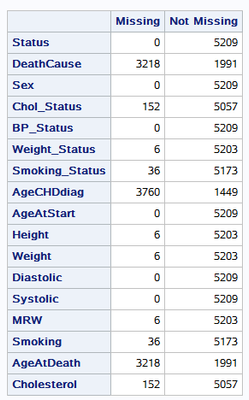## proc iml for counting missing values for all variables in a dataset.

Hi Everyone,

I am a beginner to SAS and have been using the following code to count missing values for all variables in a dataset. However, this code is printing the output in a horizontal table. I need to get in a vertical table as I have too many variables but not sure how to make that possible. By vertical I mean getting the variables as rows instead of columns. Thank you for the help in advance.

``````proc iml;
use MBS4;
read all var _NUM_ into x[colname=nNames];
n = countn(x,"col");
nmiss = countmiss(x,"col");

read all var _CHAR_ into x[colname=cNames];
close MBS4;
c = countn(x,"col");
cmiss = countmiss(x,"col");

/* combine results for num and char into a single table */
Names = cNames || nNames;
rNames = {"    Missing", "Not Missing"};
cnt = (cmiss // c) || (nmiss // n);
print cnt[r=rNames c=Names label=""];
quit; ``````
1 ACCEPTED SOLUTION

Accepted SolutionsKsharp
Super User

## Re: proc iml for counting missing values for all variables in a dataset.

``````/*Why not post it at IML forum? since it is about IML.
https://communities.sas.com/t5/SAS-IML-Software-and-Matrix/bd-p/sas_iml
*/
data MBS4;
set sashelp.heart;
run;
proc iml;
use MBS4;
read all var _NUM_ into x[colname=nNames];
n = countn(x,"col");
nmiss = countmiss(x,"col");

read all var _CHAR_ into x[colname=cNames];
close MBS4;
c = countn(x,"col");
cmiss = countmiss(x,"col");

/* combine results for num and char into a single table */
Names = t(cNames || nNames);
rNames = {"    Missing", "Not Missing"};
cnt = (cmiss`//nmiss`) || (c` // n`);
print cnt[r=Names c=rNames label=""];
quit; ``````4 REPLIES 4

## Re: proc iml for counting missing values for all variables in a dataset.

Use PROC FREQ for this:

``````proc freq data=have;
tables _all_ / missing;
run;``````

From this, play around with output datasets (OUTPUT statement or OUTPUT= option of TABLES).Ksharp
Super User

## Re: proc iml for counting missing values for all variables in a dataset.

``````/*Why not post it at IML forum? since it is about IML.
https://communities.sas.com/t5/SAS-IML-Software-and-Matrix/bd-p/sas_iml
*/
data MBS4;
set sashelp.heart;
run;
proc iml;
use MBS4;
read all var _NUM_ into x[colname=nNames];
n = countn(x,"col");
nmiss = countmiss(x,"col");

read all var _CHAR_ into x[colname=cNames];
close MBS4;
c = countn(x,"col");
cmiss = countmiss(x,"col");

/* combine results for num and char into a single table */
Names = t(cNames || nNames);
rNames = {"    Missing", "Not Missing"};
cnt = (cmiss`//nmiss`) || (c` // n`);
print cnt[r=Names c=rNames label=""];
quit; ``````## Re: proc iml for counting missing values for all variables in a dataset.

Thank you All!! All of these are working well. I just went with @Ksharp's as it gave me more compact table.

## Re: proc iml for counting missing values for all variables in a dataset.

An alternate:

```proc format;
value m
. = 'Missing'
other='Not missing'
;
value \$m
'',' '='Missing'
other='Not missing'
;
run;

proc tabulate data=sashelp.heart;
class _all_ /missing;
classlev _all_/ style=[just=r];
format _numeric_ m. _character_ \$m.;
table _all_,
n
;
run;```
From The DO Loop